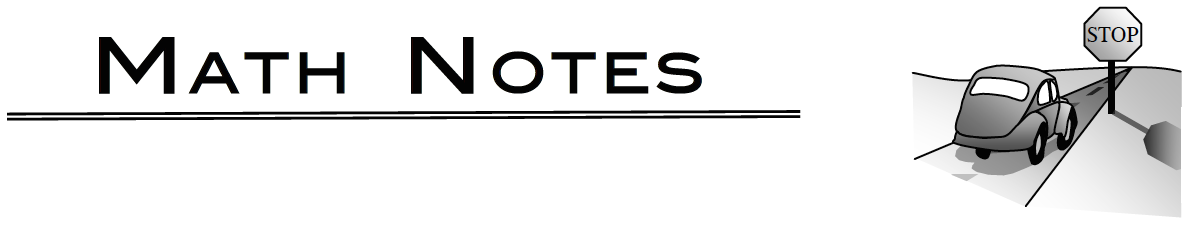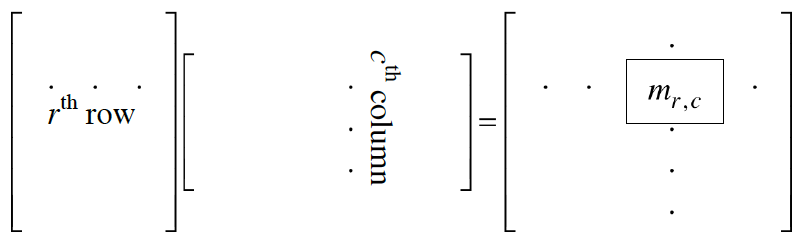### Home > PC3 > Chapter 10 > Lesson 10.1.1 > Problem10-13

10-13.

If $A = \left[ \begin{array} { c c } { 1 } & { 2 } \\ { - 3 } & { 3 } \end{array} \right]$, $B = \left[ \begin{array} { c c c } { 0 } & { 4 } & { 6 } \\ { 2 } & { - 1 } & { 5 } \end{array} \right]$, and $C = \left[ \begin{array} { l l } { 1 } & { 0 } \\ { 0 } & { 1 } \end{array} \right]$, determine each of the following matrices or write “impossible.”

If two matrices have the same dimensions, then they can be added.

1. $A + C$

1. $A + B$

1. $AB$

1. $BA$

1. $AC$

1. $CB$### Matrix Multiplication

Matrices can be multiplied as long as their dimensions “fit” correctly. That is, the number of columns in the first matrix must match the number of rows in the second. The entries of the product matrix can be obtained by calculating sums of products of rows of the first matrix with columns of the second.

To get the $m_{r,c}$ entry of the product matrix, multiply the corresponding entries in the $r^{th}$ row of the first matrix and the $c^{th}$ column of the second matrix and add the products. Visually, it looks like this:For example:

 $\begin{bmatrix} {1} & { -4 } & { 0} \\ { -2 } & { 5 } & { 3 } \end{bmatrix}$ $\begin{bmatrix} {-1} & { 0 } \\ { 2 } & { 4 } \\ { -5 } & { -3 }\end{bmatrix}$ $=\begin{bmatrix} {1(-1)+(-4)(2)+0(-5)} & { 1(0)+(-4)(4)+0(-3) } \\ { (-2)(-1)+5(2)+3(-5) } & { (-2)(0)+5(4)+3(-3) } \end{bmatrix}$ $=\begin{bmatrix} {-9} & { -16 } \\ { -3 } & { 11 } \end{bmatrix}$ $2 \times 3$ $3 \times 2$ $2 \times 2$